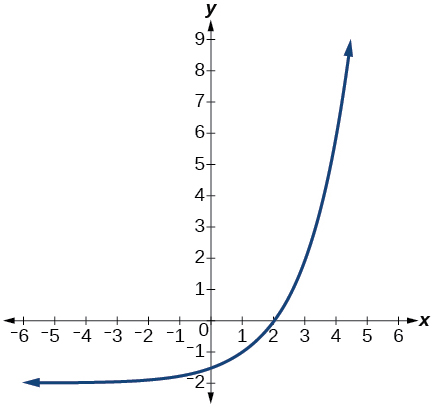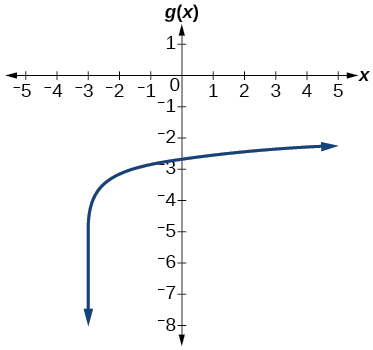# 4.8 Fitting exponential models to data  (Page 10/12)

 Page 10 / 12

The graph below shows transformations of the graph of $\text{\hspace{0.17em}}f\left(x\right)={2}^{x}.\text{\hspace{0.17em}}$ What is the equation for the transformation?## Logarithmic Functions

Rewrite $\text{\hspace{0.17em}}{\mathrm{log}}_{17}\left(4913\right)=x\text{\hspace{0.17em}}$ as an equivalent exponential equation.

${17}^{x}=4913$

Rewrite $\text{\hspace{0.17em}}\mathrm{ln}\left(s\right)=t\text{\hspace{0.17em}}$ as an equivalent exponential equation.

Rewrite $\text{\hspace{0.17em}}{a}^{-\text{\hspace{0.17em}}\frac{2}{5}}=b\text{\hspace{0.17em}}$ as an equivalent logarithmic equation.

${\mathrm{log}}_{a}b=-\frac{2}{5}$

Rewrite $\text{\hspace{0.17em}}{e}^{-3.5}=h\text{\hspace{0.17em}}$ as an equivalent logarithmic equation.

Solve for $\text{\hspace{0.17em}}x\text{\hspace{0.17em}}\text{\hspace{0.17em}}{\mathrm{log}}_{64}\left(x\right)=\frac{1}{3}\text{\hspace{0.17em}}$ to exponential form.

$x={64}^{\frac{1}{3}}=4$

Evaluate $\text{\hspace{0.17em}}{\mathrm{log}}_{5}\left(\frac{1}{125}\right)\text{\hspace{0.17em}}$ without using a calculator.

Evaluate $\text{\hspace{0.17em}}\mathrm{log}\left(\text{0}\text{.000001}\right)\text{\hspace{0.17em}}$ without using a calculator.

$\mathrm{log}\left(\text{0}\text{.000001}\right)=-6$

Evaluate $\text{\hspace{0.17em}}\mathrm{log}\left(4.005\right)\text{\hspace{0.17em}}$ using a calculator. Round to the nearest thousandth.

Evaluate $\text{\hspace{0.17em}}\mathrm{ln}\left({e}^{-0.8648}\right)\text{\hspace{0.17em}}$ without using a calculator.

$\mathrm{ln}\left({e}^{-0.8648}\right)=-0.8648$

Evaluate $\text{\hspace{0.17em}}\mathrm{ln}\left(\sqrt{18}\right)\text{\hspace{0.17em}}$ using a calculator. Round to the nearest thousandth.

## Graphs of Logarithmic Functions

Graph the function $\text{\hspace{0.17em}}g\left(x\right)=\mathrm{log}\left(7x+21\right)-4.$Graph the function $\text{\hspace{0.17em}}h\left(x\right)=2\mathrm{ln}\left(9-3x\right)+1.$

State the domain, vertical asymptote, and end behavior of the function $\text{\hspace{0.17em}}g\left(x\right)=\mathrm{ln}\left(4x+20\right)-17.$

Domain: $\text{\hspace{0.17em}}x>-5;\text{\hspace{0.17em}}$ Vertical asymptote: $\text{\hspace{0.17em}}x=-5;\text{\hspace{0.17em}}$ End behavior: as $\text{\hspace{0.17em}}x\to -{5}^{+},f\left(x\right)\to -\infty \text{\hspace{0.17em}}$ and as $\text{\hspace{0.17em}}x\to \infty ,f\left(x\right)\to \infty .$

## Logarithmic Properties

Rewrite $\text{\hspace{0.17em}}\mathrm{ln}\left(7r\cdot 11st\right)\text{\hspace{0.17em}}$ in expanded form.

Rewrite $\text{\hspace{0.17em}}{\mathrm{log}}_{8}\left(x\right)+{\mathrm{log}}_{8}\left(5\right)+{\mathrm{log}}_{8}\left(y\right)+{\mathrm{log}}_{8}\left(13\right)\text{\hspace{0.17em}}$ in compact form.

${\text{log}}_{8}\left(65xy\right)$

Rewrite $\text{\hspace{0.17em}}{\mathrm{log}}_{m}\left(\frac{67}{83}\right)\text{\hspace{0.17em}}$ in expanded form.

Rewrite $\text{\hspace{0.17em}}\mathrm{ln}\left(z\right)-\mathrm{ln}\left(x\right)-\mathrm{ln}\left(y\right)\text{\hspace{0.17em}}$ in compact form.

$\mathrm{ln}\left(\frac{z}{xy}\right)$

Rewrite $\text{\hspace{0.17em}}\mathrm{ln}\left(\frac{1}{{x}^{5}}\right)\text{\hspace{0.17em}}$ as a product.

Rewrite $\text{\hspace{0.17em}}-{\mathrm{log}}_{y}\left(\frac{1}{12}\right)\text{\hspace{0.17em}}$ as a single logarithm.

${\text{log}}_{y}\left(12\right)$

Use properties of logarithms to expand $\text{\hspace{0.17em}}\mathrm{log}\left(\frac{{r}^{2}{s}^{11}}{{t}^{14}}\right).$

Use properties of logarithms to expand $\text{\hspace{0.17em}}\mathrm{ln}\left(2b\sqrt{\frac{b+1}{b-1}}\right).$

$\mathrm{ln}\left(2\right)+\mathrm{ln}\left(b\right)+\frac{\mathrm{ln}\left(b+1\right)-\mathrm{ln}\left(b-1\right)}{2}$

Condense the expression $\text{\hspace{0.17em}}5\mathrm{ln}\left(b\right)+\mathrm{ln}\left(c\right)+\frac{\mathrm{ln}\left(4-a\right)}{2}\text{\hspace{0.17em}}$ to a single logarithm.

Condense the expression $\text{\hspace{0.17em}}3{\mathrm{log}}_{7}v+6{\mathrm{log}}_{7}w-\frac{{\mathrm{log}}_{7}u}{3}\text{\hspace{0.17em}}$ to a single logarithm.

${\mathrm{log}}_{7}\left(\frac{{v}^{3}{w}^{6}}{\sqrt{u}}\right)$

Rewrite $\text{\hspace{0.17em}}{\mathrm{log}}_{3}\left(12.75\right)\text{\hspace{0.17em}}$ to base $\text{\hspace{0.17em}}e.$

Rewrite $\text{\hspace{0.17em}}{5}^{12x-17}=125\text{\hspace{0.17em}}$ as a logarithm. Then apply the change of base formula to solve for $\text{\hspace{0.17em}}x\text{\hspace{0.17em}}$ using the common log. Round to the nearest thousandth.

$x=\frac{\frac{\mathrm{log}\left(125\right)}{\mathrm{log}\left(5\right)}+17}{12}=\frac{5}{3}$

## Exponential and Logarithmic Equations

Solve $\text{\hspace{0.17em}}{216}^{3x}\cdot {216}^{x}={36}^{3x+2}\text{\hspace{0.17em}}$ by rewriting each side with a common base.

Solve $\text{\hspace{0.17em}}\frac{125}{{\left(\frac{1}{625}\right)}^{-x-3}}={5}^{3}\text{\hspace{0.17em}}$ by rewriting each side with a common base.

$x=-3$

Use logarithms to find the exact solution for $\text{\hspace{0.17em}}7\cdot {17}^{-9x}-7=49.\text{\hspace{0.17em}}$ If there is no solution, write no solution .

Use logarithms to find the exact solution for $\text{\hspace{0.17em}}3{e}^{6n-2}+1=-60.\text{\hspace{0.17em}}$ If there is no solution, write no solution .

no solution

Find the exact solution for $\text{\hspace{0.17em}}5{e}^{3x}-4=6\text{\hspace{0.17em}}$ . If there is no solution, write no solution .

Find the exact solution for $\text{\hspace{0.17em}}2{e}^{5x-2}-9=-56.\text{\hspace{0.17em}}$ If there is no solution, write no solution .

no solution

Find the exact solution for $\text{\hspace{0.17em}}{5}^{2x-3}={7}^{x+1}.\text{\hspace{0.17em}}$ If there is no solution, write no solution .

Find the exact solution for $\text{\hspace{0.17em}}{e}^{2x}-{e}^{x}-110=0.\text{\hspace{0.17em}}$ If there is no solution, write no solution .

$x=\mathrm{ln}\left(11\right)$

Use the definition of a logarithm to solve. $\text{\hspace{0.17em}}-5{\mathrm{log}}_{7}\left(10n\right)=5.$

47. Use the definition of a logarithm to find the exact solution for $\text{\hspace{0.17em}}9+6\mathrm{ln}\left(a+3\right)=33.$

$a={e}^{4}-3$

Use the one-to-one property of logarithms to find an exact solution for $\text{\hspace{0.17em}}{\mathrm{log}}_{8}\left(7\right)+{\mathrm{log}}_{8}\left(-4x\right)={\mathrm{log}}_{8}\left(5\right).\text{\hspace{0.17em}}$ If there is no solution, write no solution .

Use the one-to-one property of logarithms to find an exact solution for $\text{\hspace{0.17em}}\mathrm{ln}\left(5\right)+\mathrm{ln}\left(5{x}^{2}-5\right)=\mathrm{ln}\left(56\right).\text{\hspace{0.17em}}$ If there is no solution, write no solution .

$x=±\frac{9}{5}$

The formula for measuring sound intensity in decibels $\text{\hspace{0.17em}}D\text{\hspace{0.17em}}$ is defined by the equation $\text{\hspace{0.17em}}D=10\mathrm{log}\left(\frac{I}{{I}_{0}}\right),$ where $\text{\hspace{0.17em}}I\text{\hspace{0.17em}}$ is the intensity of the sound in watts per square meter and $\text{\hspace{0.17em}}{I}_{0}={10}^{-12}\text{\hspace{0.17em}}$ is the lowest level of sound that the average person can hear. How many decibels are emitted from a large orchestra with a sound intensity of $\text{\hspace{0.17em}}6.3\cdot {10}^{-3}\text{\hspace{0.17em}}$ watts per square meter?

how fast can i understand functions without much difficulty
what is set?
a colony of bacteria is growing exponentially doubling in size every 100 minutes. how much minutes will it take for the colony of bacteria to triple in size
I got 300 minutes. is it right?
Patience
no. should be about 150 minutes.
Jason
It should be 158.5 minutes.
Mr
ok, thanks
Patience
100•3=300 300=50•2^x 6=2^x x=log_2(6) =2.5849625 so, 300=50•2^2.5849625 and, so, the # of bacteria will double every (100•2.5849625) = 258.49625 minutes
Thomas
what is the importance knowing the graph of circular functions?
can get some help basic precalculus
What do you need help with?
Andrew
how to convert general to standard form with not perfect trinomial
can get some help inverse function
ismail
Rectangle coordinate
how to find for x
it depends on the equation
Robert
yeah, it does. why do we attempt to gain all of them one side or the other?
Melissa
whats a domain
The domain of a function is the set of all input on which the function is defined. For example all real numbers are the Domain of any Polynomial function.
Spiro
Spiro; thanks for putting it out there like that, 😁
Melissa
foci (–7,–17) and (–7,17), the absolute value of the differenceof the distances of any point from the foci is 24.
difference between calculus and pre calculus?
give me an example of a problem so that I can practice answering
x³+y³+z³=42
Robert
dont forget the cube in each variable ;)
Robert
of she solves that, well ... then she has a lot of computational force under her command ....
Walter
what is a function?
I want to learn about the law of exponent
explain this

#### Get Jobilize Job Search Mobile App in your pocket Now!ByByBy RhodesBy Robert MurphyBy Sarah WarrenBy OpenStaxBy Richley CrapoBy OpenStaxBy OpenStaxBy Michael NelsonBy Madison ChristianBy OpenStax